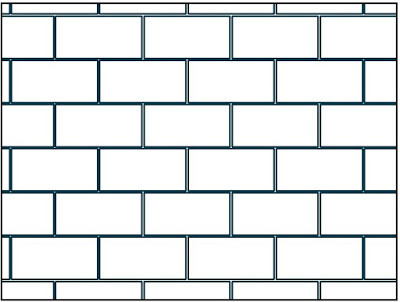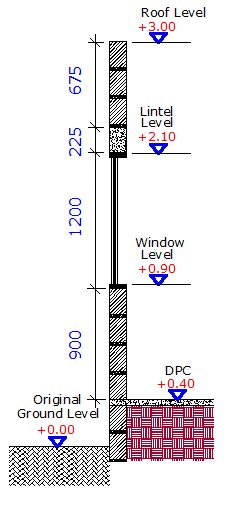Home Construction How to Calculate the Number of Blocks Required to Complete a 3...

# How to Calculate the Number of Blocks Required to Complete a 3 Bedroom FlatThe floor plan of a three bedroom flat is as shown below. It is required to estimate the quantity of 6 inches block (without holes) required to complete the project.The data from the building is as shown below;

DOORS
Door type 1 (D1) = 1 piece (Dimension = 2100 × 1200)
Door type 2 (D2) = 6 pieces (Dimension = 2100 × 900)
Door type 3 (D3) = 4 pieces (Dimension = 2100 × 700)

WINDOWS
Window Type 1 (W1) = 12 pieces (Dimension = 1200 × 1200)
Window Type 2 (W2) = 4 pieces (Dimension = 600 × 600)

Storey height = 3000mm

Foundation details of the bungalow is given below.A section through the wall of the building is given below;We are going to carry out the calculation in three main phases of construction of bungalow which are;

• Foundation to DPC
• DPC to lintel level, and
• Lintel level to overhead level.

Estimation of number blocks
The simplest approach is to estimate the number of blocks required in a metre square of wall.

The planar dimensions of a standard block in Nigeria is (450mm × 225mm).Therefore the planar area of 1 block = (0.225 × 0.45) = 0.10125 m2

Therefore one metre square of wall will contain;
1/0.10125 = 9.876

Therefore, one metre square of wall will contain approximately 10 blocks.

PHASE 1: FOUNDATION TO DPC
At this stage, the total length of the walls of the building and the height of the walls are considered. There are no openings, and the entire foundation excavation length is considered. For the building we are considering, the foundation layout is as shown below;Using any method of your choice, you can calculate the total length of the walls at the foundation level.
This is approximately equal to 100256 mm = 100.256m from my calculations.

The height of the wall from foundation to DPC (ignoring thickness of mortar) = 900mm = 0.9m

Therefore, the total area of wall = 100.256m ×  0.9m = 90.2304m2

If 10 blocks is required for 1m2 of wall, therefore (10 × 90.2304) = 903 blocks is required for 90.2304m2 of wall.

The number of blocks required to raise the building from foundation to DPC = 903 blocks (disregarding wastage and damages).

PHASE 2: DPC TO LINTEL LEVEL
At this level, we will consider the effects of openings in the buildings which includes the doors, windows, and other openings as specified in the drawing. These openings are seen at the verandah areas, and some of the entrances to the rooms. The same process of calculating the total length of walls is also adopted, after which we minus the area of the openings. This process is shown below;

Total Length of walls (excluding areas with no walls like verandahs) = 87420 mm = 87.42m

Height of wall = 2100 = 2.1m
Therefore area of wall = (87.42 × 2.1) = 183.582 m2

Total area of all doors from building data = 19.74 m2
Total area of all windows from building data = 18.72 m2

Therefore the net area of walls = 183.582 m2 – 19.74 m2 – 18.72 m2 = 145.122 m2

Hence, the total number of blocks required to take the building from DPC to lintel level = 145.122 × 10 = 1451.22 = 1452 blocks (disregarding damages and wastage)

PHASE 3: LINTEL LEVEL TO OVERHEAD LEVEL
Here, we are going to assume that the building will be chained at the lintel level (this means that the entire level of the building will be casted and reinforced with steel at the lintel level. This offers the advantage of helping the building behave as a unit, and helps in reducing cracking in the building).

Obviously, the length of wall that will be employed will not consider openings, so we are going to make use of 100.256m as calculated from the foundation level.

Hence, length of wall = 100.256m
Height of wall = 675mm = 0.675m

Therefore area of wall = (100.256 × 0.675) = 67.6728 m2

Hence, the total number of blocks required to take the building from lintel level to roof level = 67.6728 × 10 = 676.728 = 677 blocks (disregarding damages and wastage).

Ultimately, the total number of blocks required to complete the construction = 903 + 1452 + 677 = 3032 blocks (disregarding wastage).

To consider wastage, you can make a 20% increase, so that the number of blocks to be moulded or bought = 1.2 × 3032 = 3638 blocks. However when you are moulding and can guarantee the quality of blocks to be moulded, 10% increase will be satisfactory for the job.

How to estimate the quantity of materials required for moulding blocks.
Thank you for visiting us, and God bless.

1. That's quite educative for a student like myself. Thank you.

2. God bless you real good

3.Anonymous

Nice work bro… Keep it up

4.Anonymous

Can this design fit into a half plot with enough parking space.

5. Can this design fit into a half plot with enough parking space.

6. • I’ve been browsing online more than 2 hours today, yet I never found any interesting article like yours. It’s pretty worth enough for me. In my opinion, if all web owners and bloggers made good content as you did, the internet will be a lot more useful than ever before.

• Very good 👍

7. Do u have a wiring plan for this project?

8. Knowledge is power, I commend the publisher of this site, you are truly a great mind. It's quite educative for both builders and clients. Please do keep it up.

9. Nice one. God bless

10. hahahaha I have just had interest in building …. thanks for this great piece

11. Let me say a big thank you for you have given me the design of the floor plan that suits my taste. I just give it to my builder and the construction is on going.

12. Good one, God bless you real good

13.Mosfemzy

Thank you very much Sir. Your articles are very educative. God bless you real good

14.Bukola

Thank you sir

error: